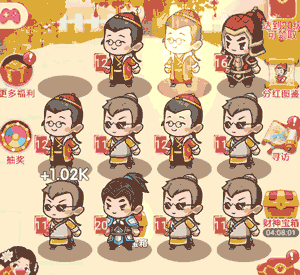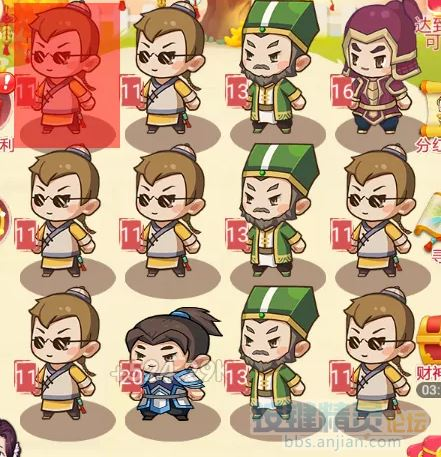1#1. For j = 0 To 2
2. For i = 0 To 3
3. //要循环的内容
4. Next
5. Nextx0+i*x,y0+j*y,x0+(i+1)*x,y0+(j+1)*y

1. For j = 0 To 2
2. For i = 0 To 3
3. //找图范围是 x0+i*x,y0+j*y,x0+(i+1)*x,y0+(j+1)*y
4. Next
5. Next

①确定有两个一样等级（数字相同）的人物，获取他们的坐标。

②把第一个人物拖动到第二个人物上。

1. For n = 6 To 10
2. FindPic 0, 0, 0, 0, "Attachment:"&n&".png","000000", 0, 0.9, intX, intY
3. If intX > -1 And intY > -1 Then
4. End If
5. Next